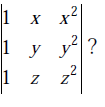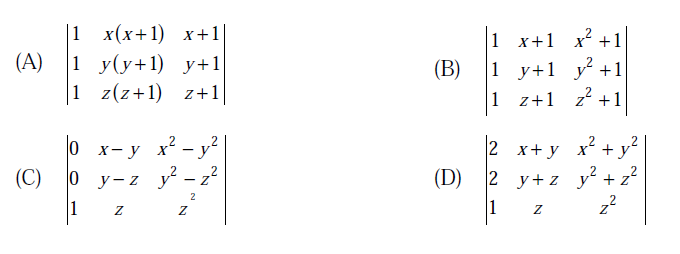Open in App
Not now

# GATE | GATE CS 2013 | Question 3

• Difficulty Level : Hard
• Last Updated : 28 Jun, 2021

Which one of the following does NOT equal to(A) A
(B) B
(C) C
(D) D

Explanation:

First of all, you should know the basic properties of determinants before approaching
For these kind of problems.
1) Applying any row or column transformation does not change the determinant
2) If you interchange any two rows, sign of the determinant will change

A = | 1 x x^2 |
| 1 y y^2 |
| 1 z z^2 |

To prove option (b)

=> Apply column transformation C2 -> C2+C1

C3 -> C3+C1

=> det(A) = | 1 x+1 x^2+1 |
| 1 y+1 y^2+1 |
| 1 z+1 z^2+1 |

To prove option (c),

=> Apply row transformations R1 -> R1-R2

R2 -> R2-R3

=> det(A) = | 0 x-y x^2-y^2 |
| 0 y-z y^2-z^2 |
| 1 z z^2 |

To prove option (d),

=> Apply row transformations R1 -> R1+R2

R2 -> R2+R3

=> det(A) = | 2 x+y x^2+y^2 |
| 2 y+z y^2+z^2 |
| 1 z z^2 |

This solution is contributed by Anil Saikrishna Devarasetty .

My Personal Notes arrow_drop_up
Related Articles